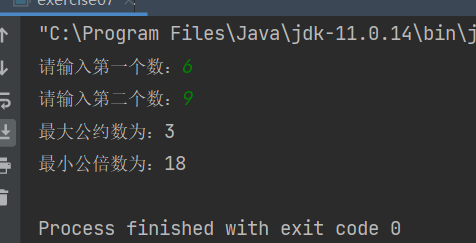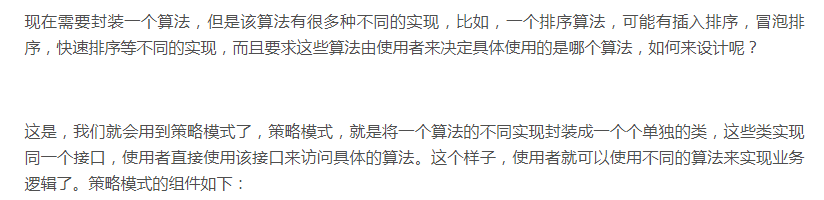+关注继续查看

# 前引：打卡！打卡！我学习了！你们呢？

# 2、数学知识：

最大公因数口诀：两个或多个整数共有约数中最大的一个。 最小公倍数：两个或多个整数公有的倍数中除零以外，最小的一个公倍数

互质的两个数的最大公因数是1，最小公倍数是这两个数的积；两个数成倍数关系时，较小的数是最大公因数，较大的是最小公倍数；一般的情况下两个数的最大公因数，用短除法，除以这两个数的公因数，直到公因数是1为止，所有除数的乘积就是最大公因数，把除数，余数全部相乘就是最小公倍数。

# 3、运行结果：# 4、代码：

### 1、两个关键解析：

        int max=0; //首先初始化为零，存公约数

//公约数从2开始，公约数的最大为两个数中最小的那个，故i<=(a1<a2?a1:a2)
//a1<a2?a1:a2 为三元运算，判断两个数的最小值
for(int i=2;i<=(a1<a2?a1:a2);i++)
{
if(a1%i==0 && a2%i==0) //如果两个数同时除尽，则为公约数
{
max=i; //由于公约数是从小到大，所以循环到最后一个便是最大公约数
}
}

        int min=0;//首先初始化为零，存公倍数

/公倍数从两个数的乘积开始，公倍数的最小为两个数中最大的那个，故j>=(a1<a2?a1:a2)
//a1<a2?a1:a2 为三元运算，判断两个数的最大值
for(int j=a1*a2;j>=(a1>a2?a1:a2);j--)
{
if(j%a1==0 && j%a2==0)  //如果同时除尽两个数，则为公倍数
{
min=j; //由于公倍数是从大到小，所以循环到最后一个便是最小公倍数
}
}

## 2、完整代码：

import java.util.Scanner;

public class exercise07 {

public static void main(String[] args) {

Scanner s1 = new Scanner(System.in);
System.out.print("请输入第一个数：");
int a1 = s1.nextInt();
System.out.print("请输入第二个数：");
int a2 = s1.nextInt();

int max=0,min=0;
for(int i=2;i<=(a1<a2?a1:a2);i++)
{
if(a1%i==0 && a2%i==0)
{
max=i;
}
}

for(int j=a1*a2;j>=(a1>a2?a1:a2);j--)
{
if(j%a1==0 && j%a2==0)
{
min=j;
}
}

System.out.println("最大公约数为："+max);
System.out.println("最小公倍数为："+min);
}
}java编程思想第四版第十一章总结

42 0java编程思想第四版第十八章总结

26 0java编程思想第四版第十章总结

19 0java编程思想第四版第七章总结

31 0java编程思想第四版第八章总结

29 0java编程思想第四版第九章总结

36 0java编程思想第四版第六章总结

32 0java编程思想第四版第五章总结

28 0Java小白踩坑录 - 使用类型擦除来实现伪泛型
Java小白踩坑录 - 使用类型擦除来实现伪泛型
20 0+关注

60

0